Analysis WebNotes
arrow_back arrow_forward

# Class Contents

## Compactness and Sequential Compactness are Equivalent

The main results of this class will show that compactness and sequential compactness are equivalent for metric spaces. The main theoretical payback from this is that, thereafter, we can read all the results from this chapter which include sequential compactness in their hypotheses as involving compactness instead.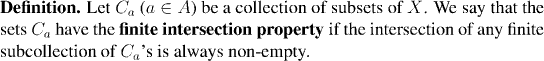We start by proving a very useful property of compact sets.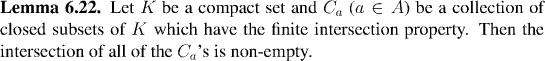Example: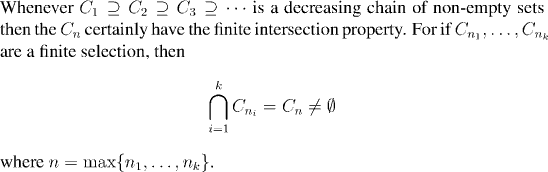Remark:

It is very important (and very easy to forget) to check that all the sets in a chain are non-empty, in order to get the finite intersection property!!

Example:

As an application of Lemma 6.22, we can give a quick proof of Proposition 6.11. Of course, the hypothesis here has to be that we are studying a continuous function on a compact rather than sequentially compact set, but once we have proved the equivalence of the two, it will amount to the same result.

Remark:

We know that sequentially compact sets are closed, so since we are going to show that sequential compactness is the same thing as compactness, we should expect that compact sets will be closed too. We show this in the next lemma.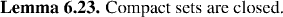We are now in a position to state the main theorem, and to begin its proof.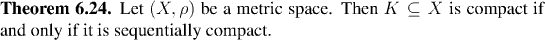As is often the case when proving equivalences, one direction of the proof is appreciably easier than the other. In this case, it is easier to prove that compact sets are sequentially compact, and this will be our first step:

## Countable and Uncountable sets

Before finishing the proof of Theorem 6.24, we need to discuss a topic from set-theory. We will explore the concepts mentioned here in more detail later in the course, but for the moment, it will be useful to be aware of an important distinction between two types of set.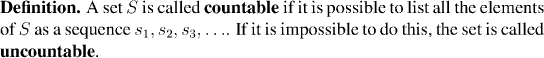At first, it isn't at all obvious that there should be any sets which are uncountable. Yet it isn't very hard to prove that the real numbers are uncountable. On the other hand (perhaps equally surprising) the rational numbers are countable.

We'll study countable and uncountable sets in more detail in Appendix A. In fact we don't explicitly need anything about countability in order to prove Theorem 6.24. The reason you need to be aware of the distinction is because, generally, when we talk about open covers of sets, there are uncountably many sets in the cover. In other words, if you have an open cover given you, there isn't any reason to expect that you can list all of the sets as a sequence, or that if you do list some of the sets as a sequence, that the sets you list will still be a cover.

The first step we take in proving that sequentially compact metric spaces are compact is to show that if we are given an arbitrary open cover of a compact set then we can always pick out a sequence of open sets from the cover, which still covers the set. In other words, we can pick out a countable cover. Then, we use that sequence of open sets to pick out a sequence of points...we apply sequential compactness...and out pops our proof!

The remainder of the proof of Theorem 6.24 is continued in the next class.